# Lecture 4: Diffusion: Fick`s second law

## Transcription

Lecture 4: Diffusion: Fick`s second law
```Lecture 4: Diffusion: Fick’s second law
Today’s topics
•
•
Learn how to deduce the Fick’s second law, and understand the basic meaning, in
comparison to the first law.
Learn how to apply the second law in several practical cases, including homogenization,
interdiffusion in carburization of steel, where diffusion plays dominant role.
In last lecture, we learned Fick’s first Law:
dc( x)
J = -D·
(Unit: D: cm2/sec; J: number/cm2/sec)
dx
Where D =
•
•
a 2ν - ΔG A /RT 1
= Γ·a2
e
6
6
Fick’s first law applies to steady state systems, where concentration keeps constant.
But in many cases of diffusion, the concentration however changes with time, how to
describe the diffusion kinetics in these cases --- demanding Fick’s second Law.
Continued from last lecture, we will learn how to deduce the Fick’s second law, and understand
the meanings when applied to some practical cases.
Let’s consider a case like this
C
dx
j(x)
A
j(x+dx)
A
x x+dx
dx
x
We can define the local concentration and diffusion flux (through a unit area, “A”) at position “x”
as:
c (x,t), J(x)
we have
[ J ( x) − J ( x + dx)]dtA
dc(x) =
,
Adx
J(x+dx) = J(x) + dJ
1
dc ( x, t )
dJ
=dt
dx
or, rewrite it in this format with “∂” replacing “d”
then, we have
∂c ( x, t )
∂J
=∂t
∂x
from the first law: J = -D·
then, we have
∂c( x)
dc( x)
= -D·
dx
∂x
∂J
∂ 2c
∂c( x, t )
==D· 2
∂t
∂x
∂x
(1)
This is the Fick’s second law.
In three-dimensional space, it can be written as:
∂c
= D · ∇ 2c .
∂t
At steady (equilibrium) state, we have
∂c( x, t ) /dt = 0 (meaning no concentration change)
Then, solving Eq. (1) gives
dc
-D· = J = constant --- back to the Fick’s first law.
dx
So, Fick’s first law can be considered as a specific (simplified) format of the second law when
applied to a steady state.
Now, let’s consider two real practical cases, and see how to solve the Fick’s second law in these
specific cases.
Case 1. Homogenization: (non-uniform à uniform)
Consider a composition profile as superimposed sinusoidal variation as shown below, where the
solid line represents the initial concentration profile (at t=0), and the dashed line represents the
profile after time τ.
2
C
t=0
β0
_
C
l
x
t=τ
At t=0,
c = c + β 0 sin
πx
l
with the Fick’s second law,
At time t,
∂c
∂ 2c
=D 2,
∂x
∂t
c(x,t) = c + β0 sin
where D is the diffusion coefficient, a constant.
π x −t τ
×e
l
Where τ = l2/π2D, τ is defined as the relaxation time.
The largest Deviation of concentration at x =
πx
l
= 1, the maximum.
, where sin
2
l
It is an exponential decay, the longer the wavelength (l), the longer the relaxation time (τ), then
the slower decay. Short wavelength dies fast. That’s why shaking always helps speed up the
dispersion, because it enables wide spreading (smaller l) of the stuff (like particles) you try to
disperse.
Case 2. Interdiffusion (the carburization of steel):doping of steel with carbon
Situation a): Doping with fixed amount of dopant
Consider a thin layer of B deposited onto A, through annealing at high temperature, we will be
able to get the concentration profile at different times, from there then we can determine the
diffusion coefficient, D
3
B
A
0
x
Infinite integration of the Fick’s second law,
∂ 2c
∂c
=D 2
∂t
∂x
We have
α
c(x,t) =
e−x
t
2
/ 4 Dt
(2)
As the B diffuses into A, the total amount of B is fixed
∫
∞
0
c( x, t )dx = N = constant
Then,
∫
∞
0
α
t
e
− x 2 / 4 Dt
dx = α 2
D
∫
∞
0
e
−(
x
2 Dt
)2
d(
To solve the above equation, let’s define y =
α2
D
since
∫
∞
0
∫
∞
0
2
x
)=N
Dt
x
then we have,
2 Dt
2
e − y dy = N
e − y dy =
π
2
then,
α=
N
πD
so Eq. (2) can now be written as
4
c(x,t) =
2
N
e − x / 4 Dt
πDt
(3)
as determined by this diffusion kinetics equation, the concentration profile of carbon at various
times will be like this
C
t3 > t2 > t1
t1
t2
t3
x
The above diffusion is one-direction (0 à +∞). But if we extends it to two-way, from -∞ to +∞
(like a droplet dissolved into a solution) with dopant at x=0, then we have
t2 > t1
t1
α= N
t2
2 πD
c (x,t) =
2
N
e− x / 4 Dt , x (-∞, ∞)
2 πDt
Situation b): Doping with a fixed surface concentration (e.g. carburization of steel)
Fe
c
CH4/CO
x=0
Carbon concentration profile shown at different times,
Carbonization thickness is defined as the diffusion depth at ½(cs+c0), which is =
Dt
Consider a real example: carbon diffusion in austenite (γ phase of steel) at 1000 °C, D=4×10-11
m2s-1, carbonization of 0.2 mm thick layer requires a time of ca. 1000 seconds, or 17 min.
5
The solution of the Fick’s second law can be obtained as follows, the surface is in contact with an
infinite long reservoir of fixed concentration of Cs. For x < 0, choose a coordinate system u.
diffuse
Cs
u
du
x=0
x
X
The fixed amount of dopant per area is Cs du=N, which diffuse toward right.
Then using Eq. (3) above, the slab “du” contributes to the concentration at x is
Cs du −u 2 / 4 Dt
e
π Dt
dc(x, t) =
So, all the slabs from u= -∞ to x totally contribute
c (x, t) =
∫
∞
dc( x, t ) = ∫
x
x
u
, then,
2 Dt
Defining y =
c (x, t) =
=
=
2c s
∫
2c s
π
π
[
∞
2
e− y dy ,
x / 2 Dt
π
2c s
2
cs
e−u / 4 Dt du
π Dt
∞
[
∫
∞
0
2
e − y dy -
π
2
= cs [ 1- erf (
-
∫
∫
x / 2 Dt
0
x / 2 Dt
0
2
e − y dy ]
2
e − y dy ]
x
)]
2 Dt
Where error function
erf (z) =
2
π
z
∫e
0
− y2
dy
6
Considering boundary conditions:
c (x = 0) = cs , constant, fixed.
c (x = ∞) = c0, corresponding to the original concentration of carbon existing in the phase, c0
remains constant in the far bulk phase at x = ∞.
c (x, t) = cs – (cs –c0)erf (
x
)
2 Dt
the concentration profile shown above follows this diffusion equation.
Now let’s consider Interdiffusion as shown below, which represents more general cases.
c1 > c2
C
c1
C1
C2
t=0
c1 + c2
2
t
c2
x=0
0
Solving the Fick’s second law gives
c (x,t) = (
x
c1 + c2
c + c2
)–( 1
) erf (
)
2
2
2 Dt
Interdiffusion is popular between two semi-infinite specimens of different compositions c1, c2,
when they are joined together and annealed, or mixed in case of two solutions (liquids). Many
examples in practice fall into the case of interdiffusion, including two semiconductor interface,
metal-semiconductor interface, etc.
7
```

### Homework 2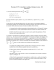More information

### Diffusion Lab Questions AP Biology Name________________________ 1. What is Kinetic Energy?More information

### Measurement of Cardiac Output in the Cath Lab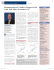More information

### Diffusion, Osmosis, Active Transport Diffusion Facilitated Diffusion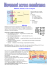More information

### Materials Kinetics Fundamentals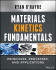More information

### DIFFUSION IN SOLIDS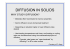More information

### QuadraTec™ - Auralex Acoustics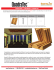More information

### L11_Solid_Diffusion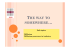More information

### Highly corrupted image inpainting through hypoelliptic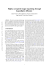More information

### May-June 2014 Terv Trails - Lone Star Belgian Tervuren Club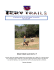More information

### Diffusion in porous media - Fritz-Haber-Institut der Max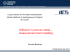More information

### Diffusion in porous media - Fritz-Haber-Institut der Max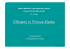More information

### NARSTCO Steel Ties Give Short Lines Longer Life.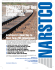More information

### ZIMA 126-CS-Fabrication.indd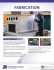More information

### Heroes and Highlights in the History of Diffusion - Qucosa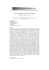More information

### Gershenson 5th Annual Golf and Fish Trip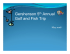More information

### Brochure SFBM.indd - Werkhuizen Hengelhoef Industrial Contracting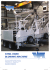More information

### Fontaine Xcalibur Extendable Flatbed Trailer Find out why more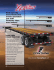More information

### Introduction to - staging.files.cms.plus.com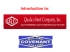More information

### PG8AC, PGAC - Drinking Fountains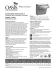More information

### practice exam 1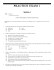More information

### Collaborative Diffusion: Programming Antiobjects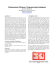More information